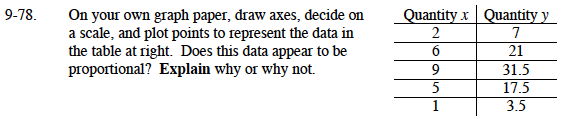### Home > MC1 > Chapter 9 > Lesson 9.2.3 > Problem9-78

9-78.Remember that proportional means that there is a multiplier you can use to find one quantity when you are given the other.
What do you multiply by x to get y? Finding the multiplier should be easier with the smaller numbers.
1(?) = 3.5
2(?) = 7
In both cases you multiply by 3.5, this is true for every x and y pair.

Explore the problem using the eTool below.
Click the link at right for the full version of the eTool: MC1 9-78 HW eTool